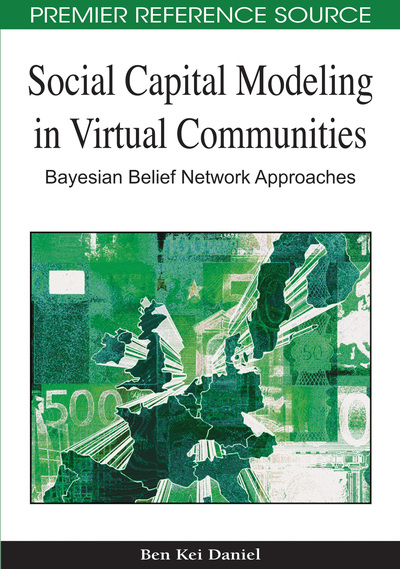# Construction of Bayesian Models

DOI: 10.4018/978-1-60566-663-1.ch011
Available
\$37.50
No Current Special Offers

## Abstract

Bayesian Belief Networks (BBNs) are increasingly used for understanding different problems in many domains. Though BBN techniques are elegant ways of capturing uncertainties, knowledge engineering effort required to create and initialize a network has prevented many researchers from using them. Even though the structure of the network and its conditional and initial probabilities could be learned from data, data is not always available and/or too costly to obtain. Furthermore, current algorithms used to learn relationships among variables, initial and conditional probabilities from data are often complex and cumbersome to employ. A qualitative Bayesian network approach was introduced to address some of the difficulties in building models that mainly depend on quantitative data. Building BBN models from quantitative data presupposes that relationships among variables or concepts of interests are known and can be correlated, causally related or they can relate to each other independently. The interdependency or relationships among the variables enable more reliable inferences which in turn help in making informed decisions about results of the model.This Chapter presents qualitative techniques and algorithms for creating Bayesian belief network models. It simplifies the construction of Bayesian models in few steps. The goal of the Chapter is to introduce the reader to the basic principles underlying the constructions of Bayesian Belief Network.
Chapter Preview
Top

## Simplifying The Construction Of Bayesian Models

For many years the process of constructing Bayesian models remained unattractive to many scientists and researchers. This is due to the complexity of the process involved and the number of expertise required, which typically is a knowledge engineer, a domain expert, a statistician and many others. In addition, the information or knowledge necessary for building Bayesian models might not be easy to obtain, as it sometimes come from more than one source (including technical manuals, test procedures, and empirical data).

Furthermore, at a minimum level, the background needed to build Bayesian models requires expertise in applied statistic and at least a reasonable knowledge of a probability theory and its inner working. The Statistical knowledge required is for initializing or creating baseline reasoning probability numbers for a model. Most of the techniques for obtaining these numbers are quantitatively driven and for the most part quite challenging to obtain.

Most recently qualitative approaches for soliciting baseline reasoning probability numbers for Bayesian models were introduced to reduce the quantitative complexity. Qualitative-based approaches are becoming popular among researchers. For at least a decade, they have helped researchers analyze complex problems and provide guidance/support for decision-making. Using the qualitative approaches, initial Bayesian models are built and are refined once appropriate data is obtained. Wellman (1990) work perhaps is the forerunner in the employment of qualitative methods. He introduced the qualitative abstraction of Bayesian Belief Network known as qualitative Bayesian networks (QBN) to help researchers and knowledge engineers overcome the problems of building a quantitative Bayesian Belief models. In other words, instead of using numerical probability distributions, a qualitative Bayesian Network approach uses the concept of positive and negative influences between variables. It first assumes an ordering relationship between variables. For example, X has a positive (+) influence on Z, if choosing a high probability value of X produces higher probability values for Z. In a similar way a negative influence between two variables is defined, that is to say if X has a negative influence on Z, so similarly choosing a negative values for X results to negative values for Z.

## Complete Chapter List

Search this Book:
Reset